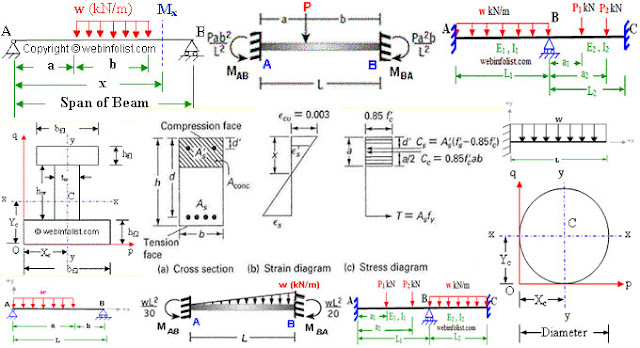How to Draw Shear Force and Bending Moment Diagram in case of Cantilever Beam

# How to Draw Shear Force and Bending Moment Diagram in case of Cantilever BeamSo in this tutorial, we are going to explain you how to draw shear force and bending moment diagram in instance of cantilever beam carrying uniformly distributed load and point load.

It is carrying a uniformly distributed load ‘UDL’ of 0.8 kilo Newton/meter at a distance of 1.5 meter from the free end and also a point load of 2 kilo Newton at a distance of 1.5 meters from the free end. Now, due to the specific downward acting rule, the reaction is RA at the point A and we have to find out this reaction. As per shear force diagram calculations, upward forces are set negative, while downward forces are set positive.

1.